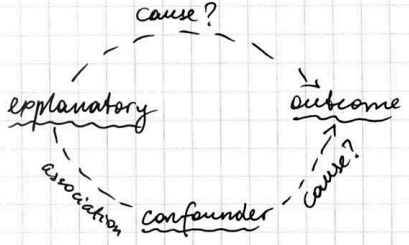# ML Wiki

## Variables in Observations

A paradigm for Observation Studies

• 2 or more group
• response variables (outcome) to be compared between the groups
• an explanatory variable is an variable that can be used to explain the differences in the response
• And we want to avoid confounding variables - variables that may affect the response variable

## Relationships

The relationships between the explanatory variable and the outcome

• causes: changes in the explanatory variables case the outcome to change
• reverse causation: changes in outcome cause the explanatory variable to change
• coincidence: just pure chance
• common cause: some other variable causes both the explanatory variables and the outcome to change
• a confounding variable is associated with the explanatory variable and causes the outcome to change
it's impossible to know whether to attribute changes in the outcome to changes in the explanatory variable or in the confounding variable

Confounding variables:### Lurking variables

Lurking variables are variables that are not considered in the analysis, but may affect the nature of the relationship between the explanatory variable and the outcome

E.g. smokers are less likely to die after 75+ years. This is not true when we consider it within age groups.

A lurking variable can be

• a confounding variable,
• or the source of the common response,
• or another variable that, when considered, changes the nature of the association

## Variables in Experiments

• Response variable (or dependent variable) - the outcome of interest, measured on each subject or entry participating in the study
• Explanatory variable (or predictor, or independent variable) - a variable that we think may help to explain the value of the response variable.

• Experiment - when a researcher manipulates the explanatory variable to see the effect on the response.

## Factors

• A categorical explanatory variable in an experiment is a factor
• The values of a factor are its levels
• A particular combination of values for the factors is called a treatment

Example:

• factor 1: $A$, $B$
• factor 2: $X$, $Y$
• 4 treatments: $A X$, $A Y$, $B X$, $X Y$

Experimental unit - the smallest using to which a treatment is applied, and a block - is a group of similar experimental units